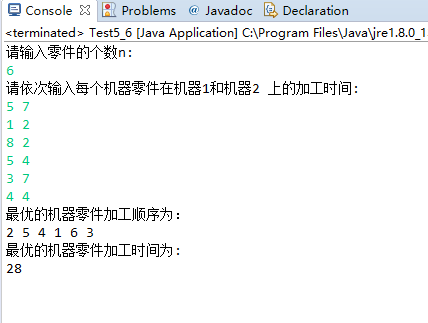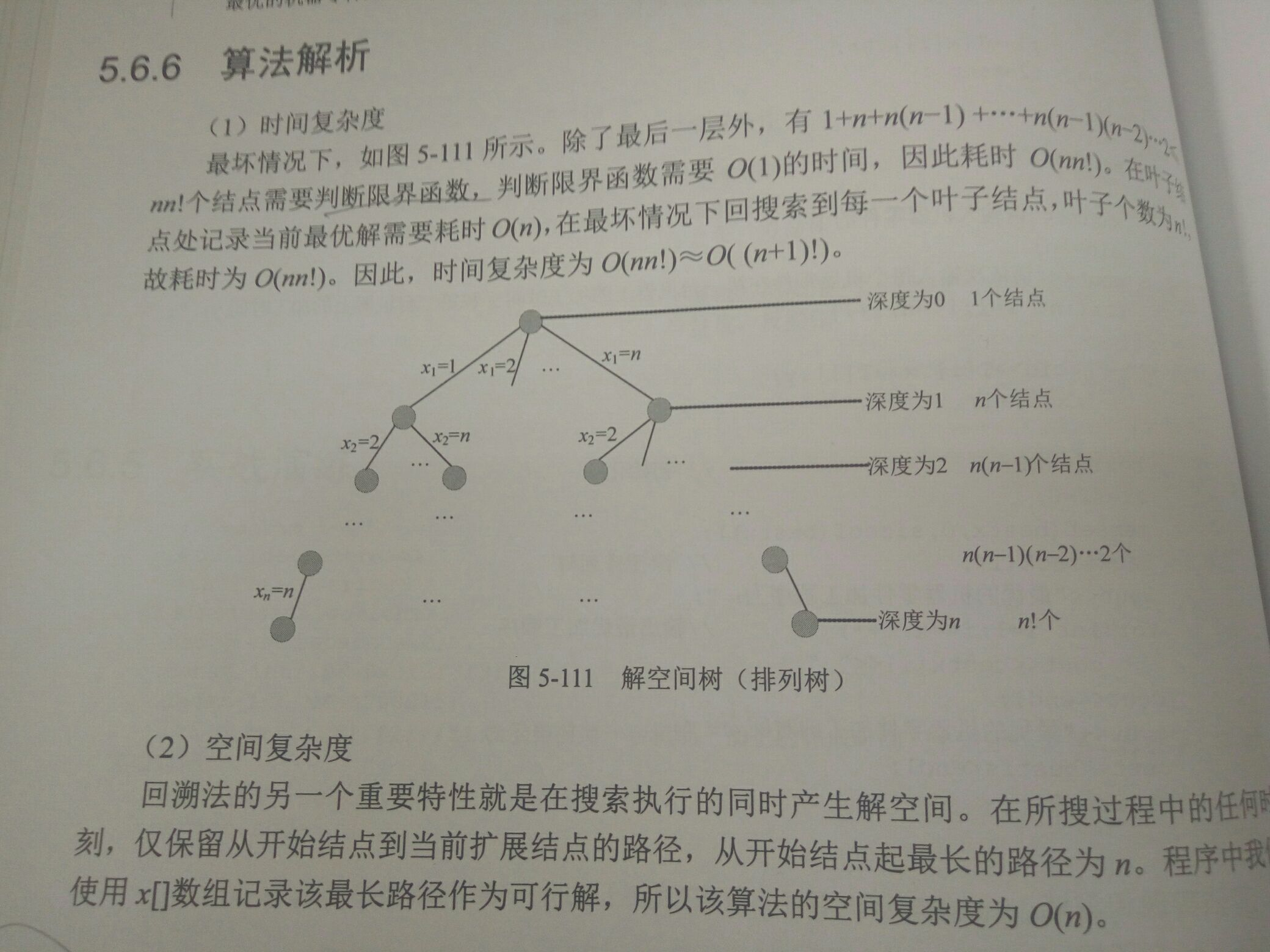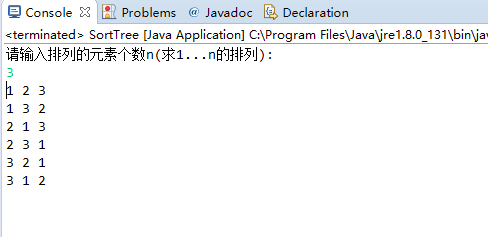# 回溯法--最优加工顺序（机器零件加工）

package com.duoduo.day316;
/**
* 最优加工顺序（机器零件加工问题）
* 问题描述：  有n个机器零件，每个零件必须先由机器1处理，再由机器2处理。零件Ji需要机器1，2处理时间为T1i,T2i.
* 			 如何安排零件加工顺序，使第一个零件从机器1上加工开始到最后一个零件在机器2上加工完成，所需的总加工时间最短？
* 问题转化：  拿实例进行分析发现：机器1可以连续加工，机器2开始的时间=max（当前机器1的下线时间f1, 机器2 的下线时间f2）
* 算法设计：  1 定义问题解空间：{x1,x2...xn}分量xi表示第i个加工的零件号 ，n个零件组成的集合为S={1,2..n},xi的取值为S-{x1,x2..X(i-1))
* 			2 解空间的组织结构： 一棵排列树   树深度为n  n!个叶子节点
* 			3 搜索解空间    约束条件-----每一种顺序均可以  -----没有约束
* 				       限界条件----f2<bestf   f2=0,bestf=无穷大（初始值）  f2:当前已完成的零件在机器2上加工结束的时间
* 																		bestf:当前找到的最优方案的加工时间
* @author 多多
*
*/
import com.duoduo.day316.*;
import java.util.Scanner;
public class Test5_6 {
static int max=100;   			//默认数组大小
static int n,bestf,f1,f2;   		// n为零件个数   bestf为最优方案加工总时间   f1当前机器1 上加工的完成时间   f2当前机器2上加工的完成时间
static int []x=new int[max];           // 记录加工的零件序号
static int[] bestx=new int[max];       // 记录最优方案零件的加工顺序
static Node [] T=new Node[max];        // 储存具体机器零件的数组

public static void main(String [] args) {
Scanner sc=new Scanner(System.in);
System.out.println("请输入零件的个数n:");
n=sc.nextInt();
System.out.println("请依次输入每个机器零件在机器1和机器2 上的加工时间:");
for(int i=1;i<=n;i++) {
T[i]=new Node();
T[i].x=sc.nextInt();      //分别给每个零件对象属性赋值
T[i].y=sc.nextInt();
x[i]=i;                   //零件序号赋值
}

//初始化
bestf=Integer.MAX_VALUE;     //赋值为最大整数
backTrack(1);                //从第一个零件开始进行深度搜索
System.out.println("最优的机器零件加工顺序为：");
for(int i=1;i<=n;i++) {
System.out.print(bestx[i]+" ");   //输出最优加工顺序
}
System.out.println();
System.out.println("最优的机器零件加工时间为:");
System.out.println(bestf);
sc.close();
}

/*深度搜素*/
private static void backTrack(int t) {
if(t>n) {                     //搜索到达叶子节点  记录可行解  和 当前最优时间
for(int i=1;i<=n;i++) {
bestx[i]=x[i];
}
bestf=f2;
return;
}

for(int i=t;i<=n;i++) {        //枚举

f1+=T[x[i]].x;             //f1一直在不间断累加
int temp1=f2;
int temp2=0;
f2=max(f1,f2)+T[x[i]].y;   //f2 在f1和f2中取最大值后+此时的机器2时间
if(f2<bestf) {            //限界条件

temp2=x[i];         //交换   全排列树
x[i]=x[t];
x[t]=temp2;

backTrack(t+1);     //继续深度搜索

temp2=x[i];         //复位 反操作
x[i]=x[t];
x[t]=temp2;
}
f1-=T[x[i]].x;          //回溯法
f2=temp1;
}

}

private static int max(int i, int j) {
return i>j?i:j;
}
}package com.duoduo.day316;
/**
* 排列树
* 所有可能的排列情况  n!种  分别列出
*
*/
import java.util.Scanner;
public class SortTree {
static int max=50;
static int n;                   //排列的元素个数
static int[] x=new int[max];    //解向量

public static void main(String [] args) {
Scanner sc=new Scanner(System.in);
System.out.println("请输入排列的元素个数n(求1...n的排列):");
n=sc.nextInt();
for(int i=1;i<=n;i++) {    //初始化
x[i]=i;
}
myArray(1);              //从1开始深度搜素排列
sc.close();
}

/*深度搜索*/
private static void myArray(int t) {
if(t>n) {
for(int i=1;i<=n;i++) {    //输出排列
System.out.print(x[i]+" ");
}
System.out.println();
return;
}

for(int i=t;i<=n;i++) {  //枚举
int temp;
temp=x[t];           //交换
x[t]=x[i];
x[i]=temp;

myArray(t+1);  //继续搜索t+1层

temp=x[t];          //回溯时反操作
x[t]=x[i];
x[i]=temp;
}
}
}ps: 每个数开头一次 递归求解剩下序列的排列 即可得到n个数的全排列

n=3

1与1交换 求（2,3)排列

2与1交换 求（1，3）排列

3与1交换 求（2，1）排列

04-0633411-101685
11-15739
07-311432
11-203584
01-21676
02-15388
04-12124
02-04101
07-15627
©️2020 CSDN 皮肤主题: 终极编程指南 设计师:CSDN官方博客